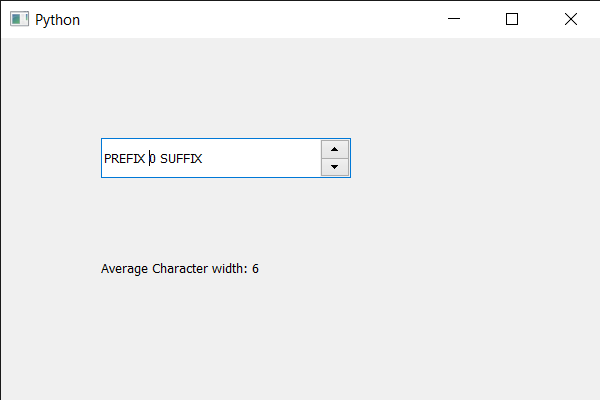Skip to content
Related Articles
PyQt5 QSpinBox – Getting average character width
• Last Updated : 26 May, 2020

In this article we will see how we can get the average character width of the spin box’s text. Average character width is basically the sum of all the character divided by the total number of character i.e the average width of glyphs in the font.

In order to do this we use `averageCharWidth` method with the spin box’s QFontMetrics object.

Syntax : font_metrics.averageCharWidth()

Argument : It takes no argument

Return : It returns integer

Below is the implementation

 `# importing libraries``from` `PyQt5.QtWidgets ``import` `*` `from` `PyQt5 ``import` `QtCore, QtGui``from` `PyQt5.QtGui ``import` `*` `from` `PyQt5.QtCore ``import` `*` `import` `sys`` ` ` ` `class` `Window(QMainWindow):`` ` `    ``def` `__init__(``self``):``        ``super``().__init__()`` ` `        ``# setting title``        ``self``.setWindowTitle(``"Python "``)`` ` `        ``# setting geometry``        ``self``.setGeometry(``100``, ``100``, ``600``, ``400``)`` ` `        ``# calling method``        ``self``.UiComponents()`` ` `        ``# showing all the widgets``        ``self``.show()`` ` `        ``# method for widgets``    ``def` `UiComponents(``self``):``        ``# creating spin box``        ``self``.spin ``=` `QSpinBox(``self``)`` ` `        ``# setting geometry to spin box``        ``self``.spin.setGeometry(``100``, ``100``, ``250``, ``40``)`` ` `        ``# setting range to the spin box``        ``self``.spin.setRange(``0``, ``999999``)`` ` `        ``# setting prefix to spin``        ``self``.spin.setPrefix(``"PREFIX "``)`` ` `        ``# setting suffix to spin``        ``self``.spin.setSuffix(``" SUFFIX"``)`` ` `        ``# creating a label``        ``label ``=` `QLabel(``self``)`` ` `        ``# making label multi line``        ``label.setWordWrap(``True``)`` ` `        ``# setting geometry to the label``        ``label.setGeometry(``100``, ``200``, ``300``, ``60``)`` ` `        ``# getting font metrics``        ``f_metrics ``=` `self``.spin.fontMetrics()`` ` `        ``# getting average character width``        ``avg_width ``=` `f_metrics.averageCharWidth()`` ` `        ``# setting text to the label``        ``label.setText(``"Average Character width: "` `+` `str``(avg_width))`` ` ` ` `# create pyqt5 app``App ``=` `QApplication(sys.argv)`` ` `# create the instance of our Window``window ``=` `Window()`` ` `# start the app``sys.exit(App.``exec``())`

Output :Attention geek! Strengthen your foundations with the Python Programming Foundation Course and learn the basics.

To begin with, your interview preparations Enhance your Data Structures concepts with the Python DS Course. And to begin with your Machine Learning Journey, join the Machine Learning – Basic Level Course

My Personal Notes arrow_drop_up